# Obscure MDX Month: Deselecting Members In An Excel PivotTable Leads To Missing Rows

Here’s some interesting (and borderline buggy) Excel PivotTable behaviour I learned about today from Charles-Henri Sauget, as well as the workaround for it courtesy of the great Greg Galloway.

Say you have a large dimension attribute hierarchy with 200,000 members on it in SSAS MD (or the equivalent in Tabular or Power Pivot) and drop it onto the rows of an Excel PivotTable. As you would expect, you get a PivotTable with 200,000 rows in it:However if you then deselect just one member on rows like so:…you’ll find that the PivotTable does not have 199,999 rows – in Excel 2016 it only has 32,000 rows:(different versions of Excel may return different numbers of rows here, but still not the full number).

If you look at the MDX generated by Excel it consists of all of the member unique names that are still selected, and unsurprisingly it’s a gigantic query:However, it turns out you can make Excel do the sensible thing and use the Except() function to return everything apart from the deselected member by going to the Field Settings dialog and selecting “Include new items in manual filter”: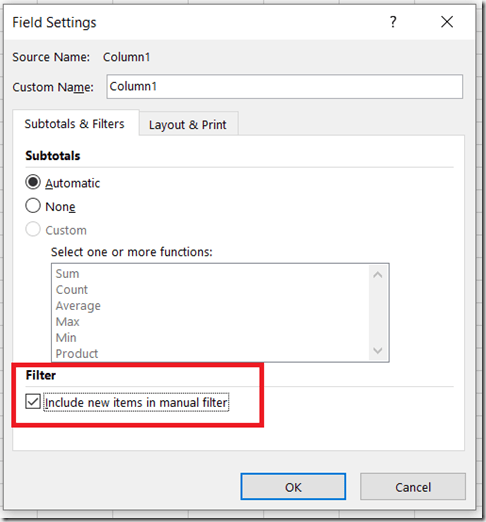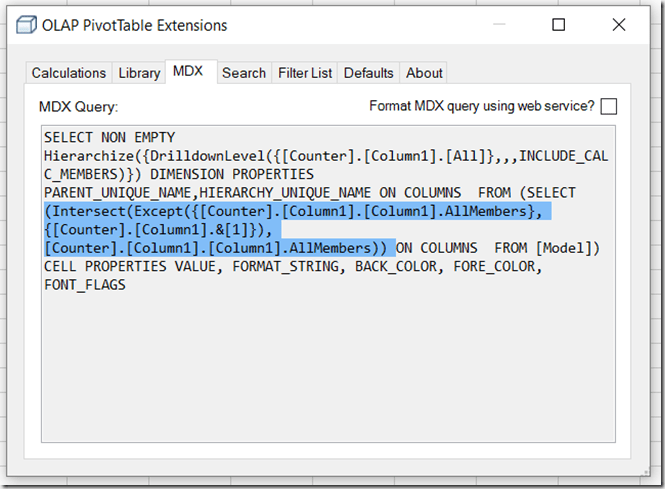This then gives you the expected number of rows in the PivotTable:I suspect the reason Excel is generating the crazy-long MDX statement by default is that it’s the only way to prevent new members being added to the PivotTable if they are added to the attribute hierarchy in future. On a really large attribute hierarchy, though, the risk is that the resulting MDX query might exceed the maximum length of a query, so Excel has to truncate the number of members returned to make the query shorter. With “Include new items in manual filter” selected, though, it’s ok if new members do get added to the PivotTable in the future so it’s ok to use the Except() function in the query.

# Obscure MDX Month: Optimising MDX That Uses The RGB() Function

In the first blog post in this series a few weeks ago I mentioned that calling Excel and VBA functions from MDX came with a query performance penalty. In this post I’ll give you an illustration of this using the VBA function that I suspect is most frequently called in MDX: the RGB() function.

Take the following MDX query as a baseline:

```WITH
MEMBER MEASURES.TEST AS
[Measures].[Internet Sales Amount]
SELECT {[Customer].[Country].[Country].MEMBERS} ON 0,
NON EMPTY
[Date].[Date].[Date].MEMBERS
*
[Product].[Product].[Product].MEMBERS
ON 1
FROM
WHERE(MEASURES.TEST)
CELL PROPERTIES
VALUE,
FORMATTED_VALUE,
BACK_COLOR
```

It returns Countries on columns and all non empty combinations of Date and Product on rows, and the calculated measure returns the value of the Internet Sales Amount measure: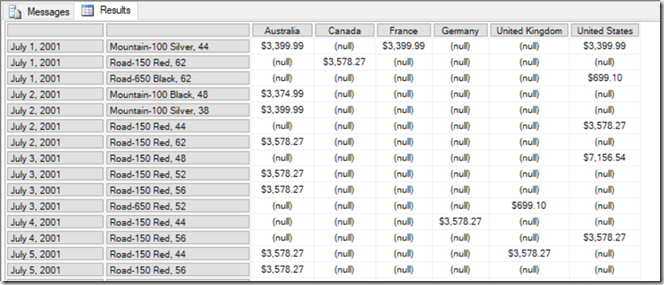On a SE engine cache it runs in 2.5 seconds on my laptop. With a BACK_COLOR property added to the calculated measure that uses the RGB() function to return the code for red if the measure value is greater than \$5000, query performance is a lot worse: it goes up to 6.5 seconds on a warm SE cache.

```WITH
MEMBER MEASURES.TEST AS
[Measures].[Internet Sales Amount]
,BACK_COLOR=
IIF([Measures].[Internet Sales Amount]>5000,
RGB(255,0,0),
RGB(255,255,255))
SELECT {[Customer].[Country].[Country].MEMBERS} ON 0,
NON EMPTY
[Date].[Date].[Date].MEMBERS
*
[Product].[Product].[Product].MEMBERS
ON 1
FROM
WHERE(MEASURES.TEST)
CELL PROPERTIES VALUE, FORMATTED_VALUE, BACK_COLOR
```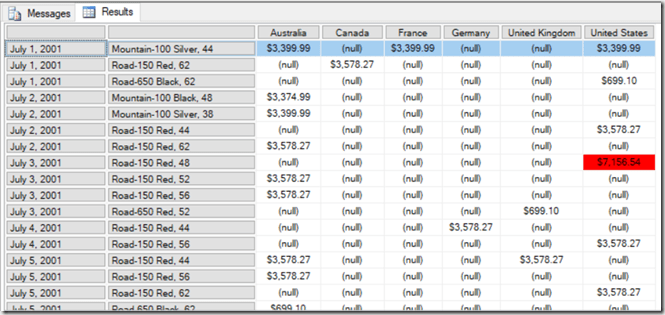That’s a big increase just to do some cell highlighting! However in this case the RGB() function can only return two possible integer values, so if you replace the RGB() function with the integers it returns, like so:

```WITH
MEMBER MEASURES.TEST AS
[Measures].[Internet Sales Amount]
,BACK_COLOR=
IIF([Measures].[Internet Sales Amount]>5000,
255,
16777215)
SELECT {[Customer].[Country].[Country].MEMBERS} ON 0,
NON EMPTY
[Date].[Date].[Date].MEMBERS
*
[Product].[Product].[Product].MEMBERS
ON 1
FROM
WHERE(MEASURES.TEST)
CELL PROPERTIES VALUE, FORMATTED_VALUE, BACK_COLOR
```

…then the query returns in around 3.5 seconds. The last thing to remember is that IIF() statements can perform better if one branch returns null, and in this case we can replace the integer value 16777215 that gives the white background with a null and get the same result:

```WITH
MEMBER MEASURES.TEST AS
[Measures].[Internet Sales Amount]
,BACK_COLOR=
IIF([Measures].[Internet Sales Amount]>5000,
255,
16777215)
SELECT {[Customer].[Country].[Country].MEMBERS} ON 0,
NON EMPTY
[Date].[Date].[Date].MEMBERS
*
[Product].[Product].[Product].MEMBERS
ON 1
FROM
WHERE(MEASURES.TEST)
CELL PROPERTIES VALUE, FORMATTED_VALUE, BACK_COLOR
```

Now the query returns in around 3 seconds, only 0.5 seconds slower than the original with no colour coding.

# Obscure MDX Month: Optimising The Performance Of Total-To-Date Calculations In SSAS Multidimensional

Here’s a SSAS Multidimensional MDX tip that I picked up at the PASS Summit back in 2008 at Mosha’s excellent “MDX Deep Dive” precon (incidentally the slides and supporting material are still available here, although a lot of the material is out of date). It’s regarding total-to-date calculations, ie calculations where you are doing a running total from the very first date you have data for up to the current date. The standard way of writing these calculations is something like this:

```WITH
MEMBER MEASURES.[TTD Sales] AS
SUM(
NULL:[Date].[Calendar].CURRENTMEMBER,
[Measures].[Internet Sales Amount])

SELECT
[Customer].[Country].[Country].MEMBERS
ON 0,
NON EMPTY
[Date].[Calendar].[Date].MEMBERS
*
[Product].[Product].[Product].MEMBERS
ON 1
FROM
WHERE(MEASURES.[TTD Sales])

```

This query runs in around 19.2 seconds on my laptop on a cold cache. However if you rewrite it like this:

```WITH
MEMBER MEASURES.[PTTD SALES] AS
SUM(
NULL:[Date].[Calendar].CURRENTMEMBER.PARENT.PREVMEMBER,
[Measures].[Internet Sales Amount])

MEMBER MEASURES.[TTD Sales] AS
MEASURES.[PTTD SALES]
+
SUM(
[Date].[Calendar].CURRENTMEMBER.FIRSTSIBLING:
[Date].[Calendar].CURRENTMEMBER,
[Measures].[Internet Sales Amount])

SELECT
[Customer].[Country].[Country].MEMBERS
ON 0,
NON EMPTY
[Date].[Calendar].[Date].MEMBERS
*
[Product].[Product].[Product].MEMBERS
ON 1
FROM
WHERE(MEASURES.[TTD Sales])

```

…it runs slightly faster: around 16.1 seconds on a cold cache on my laptop. Of course this is a very big query, and on most normal queries the difference in performance would be much less significant, but it could still be useful. In fact it’s very similar to the kind of tricks people used to optimise the performance of YTD calculations back in the days of SSAS 2000 – the subject of my second-ever blog post from December 2004! The idea here is that instead of summing up a large set of dates, the calculation sums up all the dates in the current month and then all the months from the beginning of time up to and including the previous full month. For YTD and most other something-to-date calculations trick like this are no longer needed, and indeed are counter-productive and will make your calculations slower. However it seems that for total-to-date calculations they can still help performance.

# Obscure MDX Month: Current and CurrentOrdinal

When you are writing an MDX expression, everywhere you use a set you can give that set a name and then reference the name later on. This is known as creating an inline named set, something I have blogged about a few times (see here and here) over the years. When you are iterating over a set using a function like Generate() or Filter(), if you give that set a name you can then use the Current and CurrentOrdinal functions to find out more about the item in the set returned at the current iteration.

Consider the following MDX query on the Adventure Works cube:

```SELECT
{[Measures].[Internet Sales Amount]}
ON 0,
{[Customer].[Gender].[Gender].MEMBERS
*
[Customer].[Marital Status].[Marital Status].MEMBERS}
ON 1
FROM
```

It returns a set of four tuples on rows: every combination of Gender and Marital Status:If you pass the set on rows to the Filter() function and give it a name (for example MySet) you can then use the CurrentOrdinal function to find the 1-based ordinal of the current iteration. This query uses the CurrentOrdinal function to filter the set shown above so only the first and third items in the set are returned:

```SELECT {[Measures].[Internet Sales Amount]} ON 0,
FILTER(
{[Customer].[Gender].[Gender].MEMBERS
*
[Customer].[Marital Status].[Marital Status].MEMBERS}
AS MYSET,
MYSET.CURRENTORDINAL=1 OR
MYSET.CURRENTORDINAL=3)
ON 1
FROM
```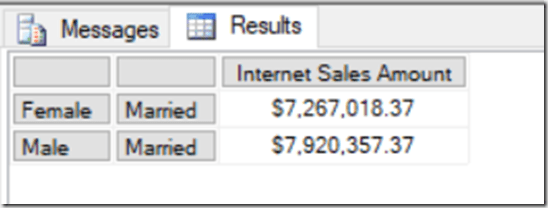With an inline named set you can also use the Current function to return the tuple at the current iteration. Here’s another query that uses the Current function to remove the tuple (Female, Single) from the set:

```SELECT
{[Measures].[Internet Sales Amount]} ON 0,
FILTER(
{[Customer].[Gender].[Gender].MEMBERS
*
[Customer].[Marital Status].[Marital Status].MEMBERS}
AS MYSET,
NOT(
MYSET.CURRENT IS
([Customer].[Gender].&[F],[Customer].[Marital Status].&[S])
)
)
ON 1
FROM
```I won’t pretend that these functions are massively useful, but fans of super-complex MDX will enjoy this vintage post where I used them.

# Obscure MDX Month: Recreating The Star Ratings Measure In MDX Using Excel Functions

I still love MDX, but I’m aware that I blog about it less and less – which is a shame, I know. Therefore I’ve decided that for the next four weeks I’m going to write about some obscure MDX topics that hopefully will make all you SSAS MD diehards out there feel less neglected… even if they don’t have much practical use.

Let’s start off with recreating my ever-popular DAX star-ratings measure in MDX. Well, not exactly pure MDX, but did you know that in MDX you can call some Excel functions (in the same way you can call some VBA functions)? It’s a really, really bad thing to do from a query performance point of view, but it does allow you to do some useful calculations that might otherwise be impossible. Here’s a query on the Adventure Works cube that uses the Excel Rept() and Unichar() functions (functions that do not exist in MDX proper) to recreate my start-ratings measure:

```WITH
MEMBER MEASURES.STARS AS
REPT(
UNICHAR(9733),
CINT([Measures].[Internet Sales Amount]/10000))
+
REPT(
UNICHAR(9734),
10-CINT([Measures].[Internet Sales Amount]/10000))

SELECT {[Measures].[Internet Sales Amount],MEASURES.STARS} ON 0,
ORDER(
[Date].[Date].[Date].MEMBERS,
[Measures].[Internet Sales Amount],
BDESC)
ON 1
FROM
```Here’s the same measure used in a PivotTable:# Power BI, SSAS Multidimensional And Dynamic Format Strings

If you’re building reports in Power BI against SSAS Multidimensional cubes then you may have encountered situations where the formatting on your measures disappears. For example, take a very simple SSAS Multidimensional cube with a single measure called Sales Amount whose FormatString property is set in SSDT to display values with a £ sign:When you build a report using the Table visualisation in Power BI Desktop using this measure, the formatted values are displayed correctly:However, if you add a SCOPE statement to the cube to alter the format string of the measure for certain cells, as in this example which sets the format string for the Sales Amount measure to \$ for Bikes:

```SCOPE([Measures].[Sales Amount], [Product].[Category].&);
FORMAT_STRING(THIS)="\$0,0.00";
END SCOPE;
```

…then you’ll find that Power BI displays no formatting at all for the measure:What’s more (and this is a bit strange) if you look at the DAX queries that are generated by Power BI to get data from the cube, they now request a new column to get the format string for the measure even though that format string isn’t used. Since it increases the amount of data returned by the query much larger, this extra column can have a negative impact on query performance if you’re bringing back large amounts of data.

There is no way of avoiding this problem at the moment, unfortunately. If you need to display formatted values in Power BI you will have to create a calculated measure that returns the value of your original measure, set the format string property on that calculated measure appropriately, and use that calculated measure in your Power BI reports instead:

```SCOPE([Measures].[Sales Amount], [Product].[Category].&);
FORMAT_STRING(THIS)="\$0,0.00";
END SCOPE;

CREATE MEMBER CURRENTCUBE.[Measures].[Test] AS
[Measures].[Sales Amount],
FORMAT_STRING="£0,0.00";
```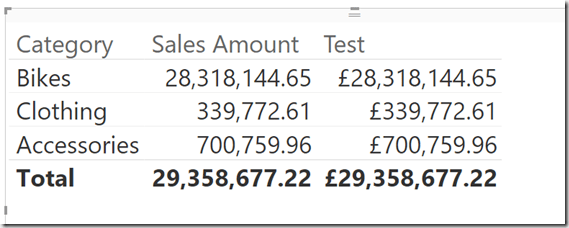Thanks to Kevin Jourdain for bringing this to my attention and telling me about the workaround, and also to Greg Galloway for confirming the workaround and providing extra details.

UPDATE October 2017: this issue appears to be fixed in the latest release of Power BI https://powerbi.microsoft.com/en-us/blog/power-bi-desktop-october-2017-feature-summary/#analytics

# Handling Missing Members In The CubeSet() Function With Power Pivot

Last week I received an email from a reader asking how to handle missing members in MDX used in the Excel CubeSet() function. My first thought was that this could be solved easily with the MDXMissingMemberMode connection string property but it turns out this can’t be used with Power Pivot in Excel 2013/6 because you can’t edit the connection string back to the Excel Data Model: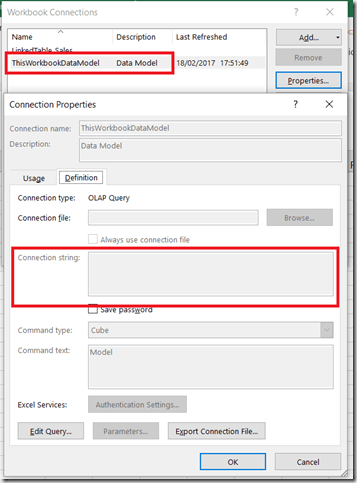Instead, you have no choice but to handle this in MDX.

Here’s an illustration of the problem. Imagine you have the following table of data on your Excel worksheet:With this table added to the Excel Data Model, you could write the following Excel formula using CubeSet():

```=CUBESET(
"ThisWorkbookDataModel",
"{[Sales].[Product].[All].[Apples],
[Sales].[Product].[All].[Oranges],
[Sales].[Product].[All].[Pears]}",
"Set")
```In the screenshot above the CubeSet() formula is used in H3, while in H4 there’s a formula using CubeSetCount() that shows the set contains three members.

If the source data is updated so that the row for Pears is deleted like so:Then the CubeSet() formula returns an error because the member Pears no longer exists:How can this be avoided? If what you actually wanted was all of the Products, whatever they were, the best thing to do is to use the MDX Members function like so:

```=CUBESET(
"ThisWorkbookDataModel",
"{[Sales].[Product].[Product].MEMBERS}",
"Set")
```

[I talk about the Members function in this post in my series of posts on MDX for Power Pivot users]

This formula does not return an error and you can see that the CubeSetCount() formula below shows the set only contains two members now:If you do need to refer to individual members then the MDX you need is more complicated:

```=CUBESET(
"ThisWorkbookDataModel",
"{[Sales].[Product].[All].[Apples],
[Sales].[Product].[All].[Oranges],
iif(
iserror(
strtomember(""[Sales].[Product].[All].[Pears]"")
),
{},
{strtomember(""[Sales].[Product].[All].[Pears]"")}
)
}",
"Set")
```This MDX uses the StrToMember() function to interpret the contents of a string as an MDX expression returning a member; if this expression returns an error then it is trapped by the IsError() function and an empty set is returned.

This isn’t particularly pretty, though, and ideally the MDXMissingMemberMode connection string property would be set to Ignore in the Excel Data Model connection string property.

# Finding Out (Approximately) How Long A Calculation Contributes To The Duration Of An MDX Query

In my last two blog posts (see here and here) I showed how to use the Calculation Evaluation and Calculation Evaluation Detailed Information trace events to work out which MDX calculations are evaluated when a query runs in Analysis Services Multidimensional. That’s very useful, but wouldn’t it be great if you could work out how long any single calculation contributes to the overall duration of a query? If you could, it would make performance tuning MDX calculations much easier.

While you can’t get an exact amount of time taken for each calculation, the good news is that it is possible to get a duration rounded to the next second if your calculation is evaluated in bulk mode.

Take a look at the following query:

```WITH

MEMBER MEASURES.DAYRANK AS
RANK(
[Date].[Date].CURRENTMEMBER,
[Date].[Date].[Date].MEMBERS)-1

IIF(
[Measures].[Internet Sales Amount]=0,
NULL,
MEASURES.DAYRANK)

MEMBER MEASURES.LASTSALERANK AS
MAX(
NULL:[Date].[Date].CURRENTMEMBER,

MEMBER MEASURES.LASTSALE AS
([Measures].[Internet Sales Amount],
[Date].[Date].[Date].MEMBERS.ITEM(MEASURES.LASTSALERANK))

MEMBER MEASURES.SIMPLECALC AS
[Measures].[Internet Sales Amount] * 2

SELECT
*
{MEASURES.SIMPLECALC, MEASURES.LASTSALE}
ON 0,
[Date].[Date].[Date].MEMBERS
ON 1
FROM

```

This query contains five calculated measures: the first four in the WITH clause, DAYRANK, HADSALE, LASTSALERANK and LASTSALE, are based on my approach for finding the last ever non-empty value for a measure across time; the final measure, SIMPLECALC, is as the name suggests a very simple calculation. On my laptop this query takes around 13 seconds to run on my laptop, on a warm Storage Engine cache. Why does it take so long? It’s clearly the calculations that are the problem, but which one(s)?

Luckily all of the calculations in this query are evaluated in bulk mode so, as I discussed in my last two posts, there is an event raised with:

Event Class = Calculation Evaluation Detailed Information

Event Subclass = 107 – RunEvalNode Finished Calculating Item

…for each of the calculations when they are evaluated. Unfortunately the Duration column for this event always shows 0, but there is a way to see approximately how long the calculation took by comparing the Start Time and Current Time columns in the trace.

The 107 – RunEvalNode event for the measure SIMPLECALC shows the same time for the Start Time and Current Time columns: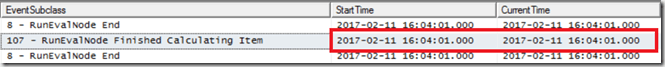This indicates that the SIMPLECALC calculation is evaluated in under a second.

However, sequence of 107 – RunEvalNode events for the LASTSALE calculation shows something different: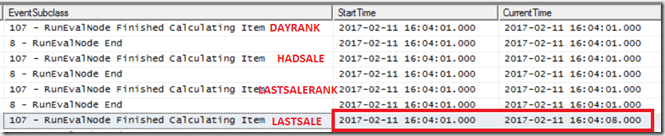There’s a gap of 7 seconds between the StartTime and the CurrentTime, and this indicates that the calculation took 7 seconds to evaluate. It’s a bit frustrating that there isn’t a way to get a more accurate duration here, but it’s still very clear which calculation is taking all the time. Even though the time for calculating LASTSALE includes the time taken for calculating LASTSALERANK, HADSALE and DAYRANK (all of which need to be calculated in order to calculation LASTSALE), the equivalent rows in the trace for these other calculations show they took under a second each. It’s only the logic inside LASTSALE itself that is slow – so that’s where any tuning needs to take place. Indeed, modifying the query to return LASTSALERANK instead of LASTSALE makes the query faster by around 6 seconds, supporting this conclusion.

If you’re curious about what the other 6 seconds of the query execution time is taken up by, it seems like it’s serialisation of the results – something I blogged about here. The query returns a cellset with 400*1190=476000 cells in, and SSAS doesn’t cope well with queries that return a large amount of data.

# Finding Out Which MDX Calculations Are Being Evaluated By Your Query In Analysis Services Multidimensional, Part 2

In part 1 of this series I showed how you can use Profiler to find out which MDX calculations are being evaluated when a query runs on SSAS Multidimensional. In this post I’ll show a practical example of why this is so useful: a situation where SSAS evaluates a calculation that isn’t needed by a query.

Do you have a Date Tool dimension (also known as a Shell dimension or Time Utility dimension) in your cube? A lot of enterprise-level SSAS cubes use this technique to allow you to write a calculation once and have it apply to multiple measures. There are two main approaches to implementing Date Tool dimensions:

• You can create a dimension with one hierarchy and one real member and then use calculated members for your calculations, or
• You can create a dimension with one hierarchy and as many real members as you need calculations, and then use SCOPE statements on these members for your calculations

The second approach, described in detail in this article, is very popular but over the years I have seen several cases where customers of mine who use it have suffered from unexplained query performance problems, problems that have been solved by using the calculated member approach instead. It turns out that the Calculation Evaluation and Calculation Evaluation Detailed Information Profiler events can shed some light on the causes of these problems.

Here’s a simple test cube with a Date Tool dimension that has three real members on it: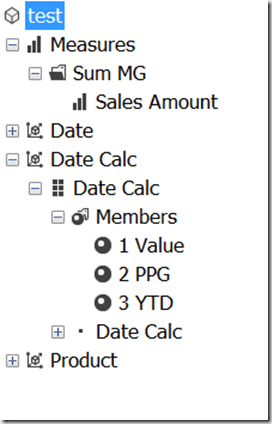Here’s the contents of the MDX Script, copied from the Calculations tab in the Cube Editor in SSDT:

```CALCULATE;

SCOPE([Date Calc].[Date Calc].&[2 PPG]);
THIS = ([Date Calc].[Date Calc].&[1 Value],
[Date].[Calendar].CURRENTMEMBER.PREVMEMBER);
END SCOPE;

SCOPE([Date Calc].[Date Calc].&[3 YTD]);
THIS = AGGREGATE(
YTD([Date].[Calendar].CURRENTMEMBER),
[Date Calc].[Date Calc].&[1 Value]);
END SCOPE;
```

As you can see, two of the members on the [Date Calc] dimension are overwritten by scoped assignments: [2 PPG] is overwritten with a previous period growth calculation and [3 YTD] is overwritten by a year-to-date calculation.

Here’s a query that includes a calculated measure defined in the WITH clause and returns two out of three of the members on the [Date Calc] dimension – but does not return the [3 YTD] calculation:

```WITH
MEMBER [Measures].QueryCalc AS
[Measures].[Sales Amount] + 1

SELECT
{
[Measures].[Sales Amount],
[Measures].QueryCalc
}
*
{
[Date Calc].[Date Calc].&[1 Value],
[Date Calc].[Date Calc].&[2 PPG]
}
ON 0,
[Date].[Calendar].[Month].MEMBERS
ON 1
FROM
TEST
```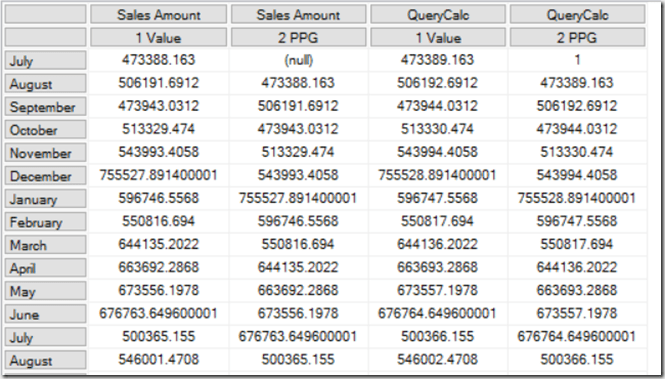Running a Profiler trace as described in my previous post reveals that when this query is run, not only are the [Query Calc] and [2 PPG] calculations evaluated, but [3 YTD] is evaluated too: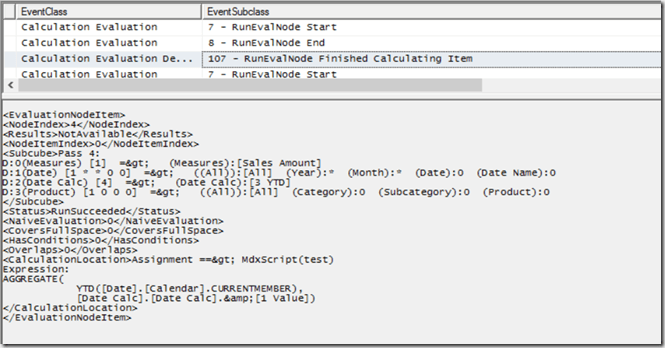It’s worth pointing out that this query was constructed deliberately to show a scenario where SSAS does decide to evaluate the [3 YTD] calculation, but in other cases it may decide otherwise. The reason it decides to do so here is due to a number of factors, including prefetching – see Jeffrey’s blog post here and the section on “Unexpected partition scans” here for some background information on this topic. Remember that in most cases prefetching is a good thing and is beneficial for performance, so if you see something like this happening in your cube you need to be sure that it’s actually causing you a performance problem before you try to prevent it.

If this is a problem for you there are a few things you can do. Rewriting your query to use subselects (if you have control over the MDX query that is being used) is one option:

```WITH
MEMBER [Measures].QueryCalc AS
[Measures].[Sales Amount] + 1

SELECT
{
[Measures].[Sales Amount],
[Measures].QueryCalc
}
*
{
[Date Calc].[Date Calc].MEMBERS
}
ON 0,
[Date].[Calendar].[Month].MEMBERS
ON 1
FROM
(SELECT
{
[Date Calc].[Date Calc].&[1 Value],
[Date Calc].[Date Calc].&[2 PPG]
}
ON 0
FROM
TEST)
CELL PROPERTIES VALUE
```

Using the following connection string properties also works, because it turns off prefetching:

```disable prefetch facts=true; cache ratio=1
```

…but as I said, this might hurt query performance in other ways.

Finally, as I said, using calculated members on your Date Tool dimension instead of the real members/scope statements approach will also work too. In my opinion this is the best solution since the problems with calculated member selection in Excel that caused problems for the calculated member Date Tool approach in the past were fixed a long time ago, and it will work even if you can’t change how your MDX queries are generated.

# Finding Out Which MDX Calculations Are Being Evaluated By Your Query In Analysis Services Multidimensional, Part 1

Since Analysis Services 2012 there have been two trace events that provide a lot of information about what’s going on in the Formula Engine when you run a query in Analysis Services Multidimensional: Calculation Evaluation and Calculation Evaluation Detailed Information. The problem is that they are not properly documented anywhere and they provide so much information that it’s difficult to interpret what they are telling you. This post on Thomas Ivarrsson’s blog (which I strongly advise you to read before you carry on) with information provided by Akshai Mirchandani of the dev team  is the only place that has any details about them and unfortunately it’s by no means comprehensive.

I don’t have the knowledge to provide a full description of these two trace events, so instead in this series of posts I want to do something less ambitious but hopefully still useful: show how you can use them to find out which MDX calculations are being evaluated when you run a query, which is of course going to be useful if you are trying to tune that query. It’s not always as easy as you might think to work out which calculations are referenced by a query: for example financial cubes often have hundreds of calculated members and/or scoped assignments, many of which are dependent on other calculations.

Here’s a super-simple example to start off with. Imagine you have a cube with just one regular measure, Sales Amount, and just one calculated measure with the following definition:

```CREATE MEMBER
CURRENTCUBE.MEASURES.[Sales Forecast] AS
[Measures].[Sales Amount] * 2;

```

Now, consider the following query:

```WITH
MEMBER MEASURES.X as 123
SELECT
{[Measures].[Sales Forecast]}
ON 0
,
[Date].[Date].[Date].MEMBERS
ON 1
FROM
[test]
CELL PROPERTIES VALUE

```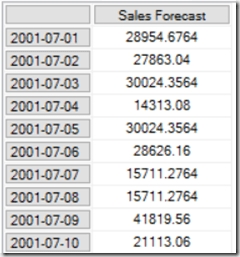The query returns the Sales Forecast calculated measure on columns and every member on the Date level of the Date hierarchy on rows – so not all that interesting. However there are two things to point out:

• The WITH clause has a calculated measure that isn’t used in the query. The reason I’ve put this in the query is to stop the Formula Engine from caching the results of any MDX calculations for longer than the lifetime of the query (see here for more details); it doesn’t affect the Storage Engine cache however. This means that every time the query is run you know that all the calculations will be evaluated and that you’ll be able to see any related activity in Profiler, and that you can run the query on a warm Storage Engine cache and won’t see many Storage Engine-related events.
• The CELL PROPERTIES clause only returns the VALUE property and not the FORMAT_STRING property which is normally returned as well. This reduces the number of Calculation Evaluation events that are raised in Profiler when the query runs and makes it easier to see the important information.

With a Profiler trace that includes the Calculation Evaluation and Calculation Evaluation Detailed Information events, when you run the query above you’ll see this: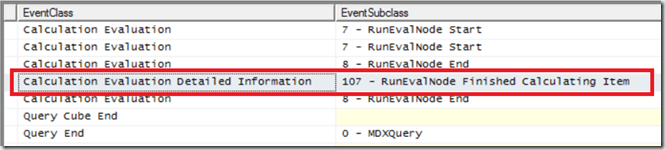There are a lot of events generated in the trace even for this simple query, but the important thing to look for is the line highlighted in the screenshot above: a Calculation Evaluation Detailed Information event with the following event subclass:

107 – RunEvalNode Finished Calculating Item

Any time you see this event you know that a calculation has been evaluated in bulk mode for a subcube (ie an area of cells) in your cube. You may see more than one RunEvalNode event for the same calculation in the same query if it was evaluated for more than one subcube.

The contents of the TextData column (which is displayed in the lower half of the screen in Profiler) for the RunEvalNode event in the trace shown above are as follows:As you can see, it tells you the MDX expression that has been evaluated for the subcube. It also tells you the name of the calculated measure, but it’s the MDX expression that’s important here because scoped assignments that overlap with a single calculated measure could mean that many different MDX expressions must be evaluated for that calculated measure.

Now for the bad news: you won’t see a RunEvalNode event for any calculations that are evaluated in cell-by-cell mode. You probably know that inefficient or badly-written calculations are often evaluated in cell-by-cell mode, which is usually slower than bulk mode, but there are cases where the Formula Engine evaluates a perfectly good calculation in cell-by-cell mode because it’s the right thing to do. For example, take a look at the following query:

```WITH
MEMBER MEASURES.X as 123
SELECT
{[Measures].[Sales Forecast]}
ON 0
FROM
[test]
CELL PROPERTIES VALUE

```It’s basically the same query as the one above but with the Rows axis removed, so it only returns a single cell. In Profiler you won’t see a RunEvalNode event because in this case the Sales Forecast calculation is evaluated in cell-by-cell mode.

That said you will see other events relating to the evaluation node for the Sales Forecast calculation, such as the Calculation Evaluation event shown here, the last for this node (NodeIndex=0, the same value that is shown in the IntegerData column) in the trace: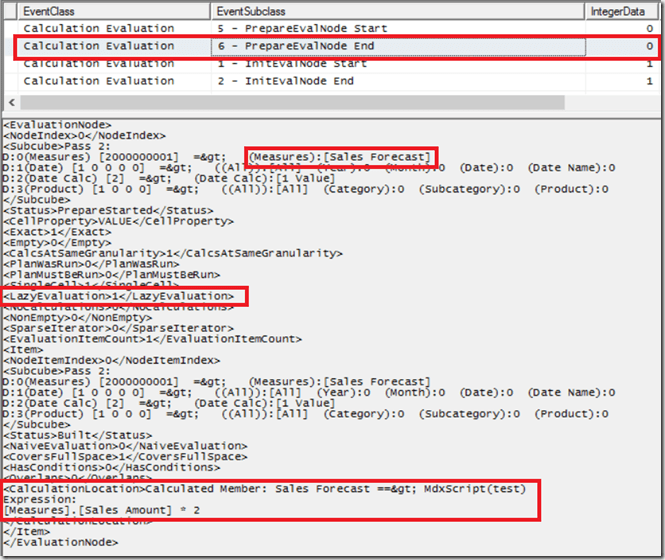Notice also the LazyEvaluation tag which is 1, which indicates a calculation that is evaluated in cell-by-cell mode.

So, to sum up, there are two ways to see which calculations are referenced by your query. With a Profiler trace and that includes the Calculation Evaluation and Calculation Evaluation Detailed Information events:

1. If your calculation is evaluated in bulk mode you will see a Calculation Evaluation Detailed Information event with the Event Subclass 107 – RunEvalNode Finished Calculating Item.
2. If your calculation is evaluated in cell-by-cell mode you will see Calculation Evaluation events for the Init-Build-Prepare stages of the evaluation node.

In the next post in this series I’ll look at a more complex scenario that shows some unexpected behaviour by SSAS.

[I am extremely grateful to Akshai Mirchandani for answering a lot of questions relating to this topic. If you want to learn more about the internals of the Formula Engine there are two other useful resources: this post by Jeffery Wang, also of the dev team, and chapter 29 of the book “Microsoft SQL Server 2008 Analysis Services Unleashed”]